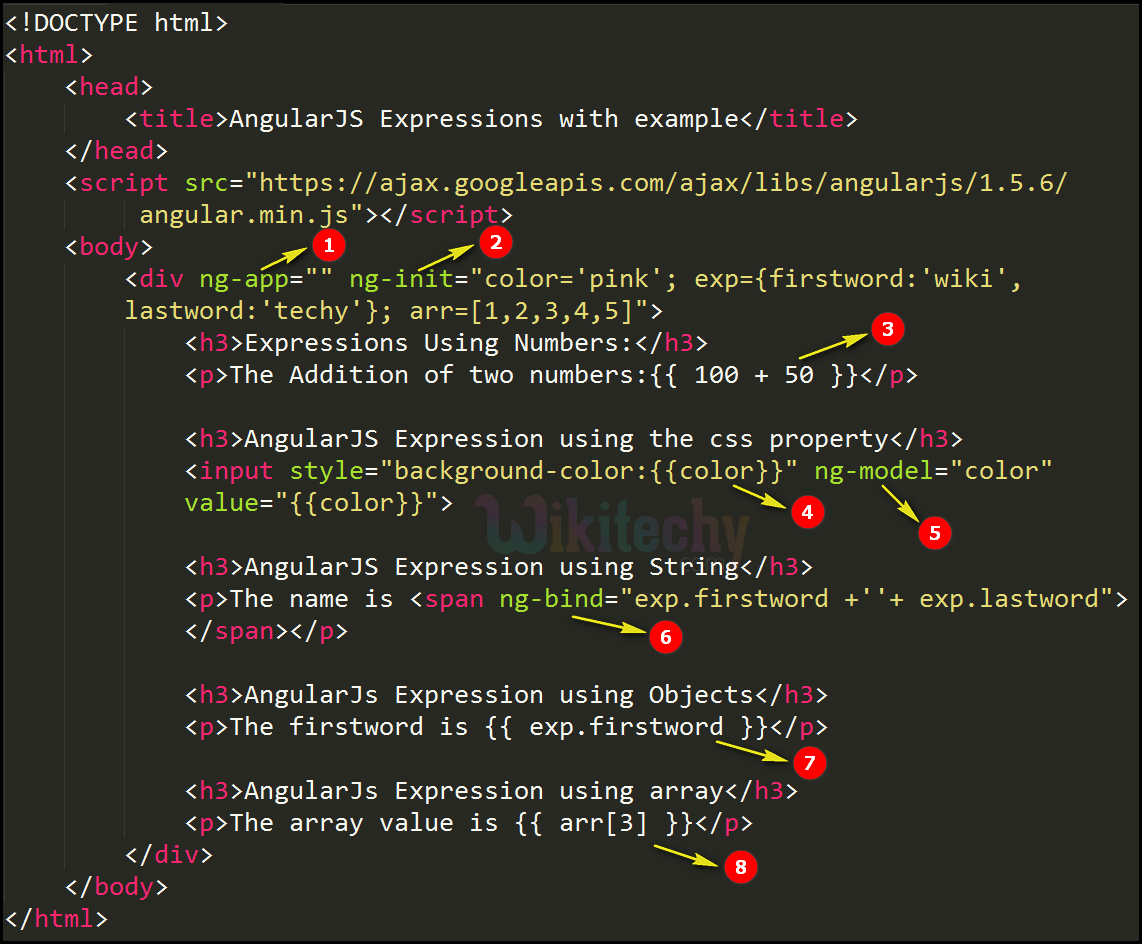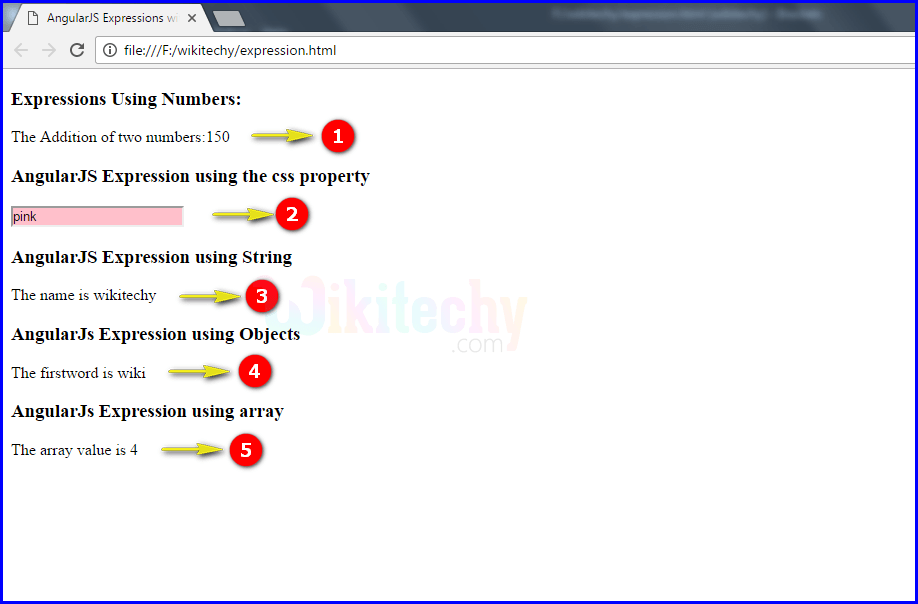# AngularJS Expressions

• AngularJS expressions are used to bind data in HTML.
• AngularJS execute the expressions and return the values where the expression is defined.
• AngularJS expressions are same as JavaScript expressions. It can be used Array, Objects, String and Number.
• 1+2 – Number.
• Hello+World - String
• a.name - Objects
• items[index] - Array
• AngularJS expressions are written inside two curly braces { { } } otherwise we can use ng-bind directives.

## AngularJs Expressions:

• AngularJS expressions does not support the conditionals, loops and exceptions.
• It support the filters.
• It does not use the function declaration, even inside the ng-init directive.
• Bitwise, comma, void, new operator and regular expression does not supported in AngularJS Expression.
• In AngularJS ignore the undefined or null properties in expression.
• Angular expressions are evaluated belongs to scope object

## Javascript Expressions:

• Javascript expressions support the conditionals, loops and exceptions.
• It does not support the filters.
• Javascript expressions use the function declaration.
• Operators are used in Javascript expressions.
• Javascript undefined properties creates ReferenceError or TypeError
• Javascipt expressions are evaluated belongs to global window.

## AngularJS Expressions using Numbers:

• The expression 100+50 executed

## AngularJS Expressions using the CSS Property:

• Controller logic for the AngularJS application.

## AngularJS Expressions using String:

• The ng-bind is used to bind the expression values to the HTML Document.

## AngularJS Expressions using Objects:

• The expression exp.firstword is get the value of the firstword from the object

## AngularJS Expressions using Array:

• The expression arr will get the 4 as a value from an array (like arr=1, arr=2, arr=3, arr=4, arr=5])

## Code Explanation for AngularJS Expression:1. The ng-app specifies the root element <div> to define AngularJS application.
2. The ng-init directive used to initialize the variables (color='pink'; exp={ firstword:'wiki', lastword:'techy' }; arr=[1,2,3,4,5]).
3. The expression 100+50 executed and the output will be updated in <p> tag.
4. The expression color (AngularJS variable) executed and the output will be updated inside the style attribute in <input> tag.
5. The ng-model bind an input field value to AngularJS application variable (“color”).
6. The ng-bind is used to bind the expression ("exp.firstword +''+ exp.lastword") values to the HTML Document.
7. The expression exp.firstword is get the value of the firstword from the object (exp) and that will be updated in the <p> tag.
8. The expression arr will get the 4 as a value from an array (like arr=1, arr=2, arr=3, arr=4, arr=5]) and the output will displayed in the <p> tag.

## Sample output:1. The output displays the addition of two numbers is 150.
2. The output shows the default background color as pink when you change the color name it will be taken as value and will change the background color automatically.
3. The output displays the concatenation of two strings “wiki” and ”techy”.
4. The output displays the firstword “wiki” by the exp object.
5. The output displays the array value 4.